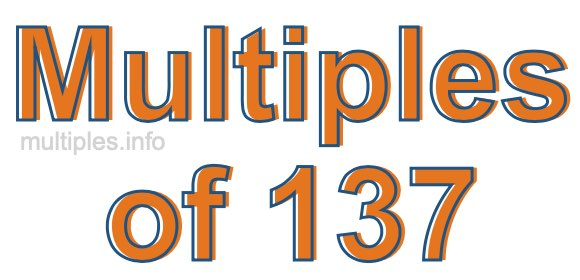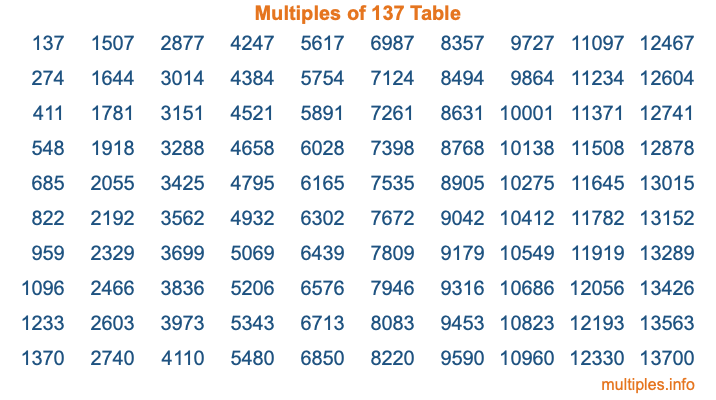Multiples of 137Welcome to the Multiples of 137 page. Here we will first teach you everything you will ever need to know about the multiples of 137, and then give you a study guide summary of everything we taught you to make sure you remember it all. Use this page to look up facts and learn information about the multiples of 137. This page will make you a multiples of one hundred thirty-seven expert!

Definition of Multiples of 137
Multiples of 137 are all the numbers that when divided by 137 equal an integer. Each of the multiples of 137 are called a multiple. A multiple of 137 is created by multiplying 137 by an integer.

Therefore, to create a list of multiples of 137, you start with 1 multiplied by 137, then 2 multiplied by 137, then 3 multiplied by 137, and so on for as long as you want. Thus, the list of the first five multiples of 137 is 137, 274, 411, 548, and 685. To see a larger list of multiples of 137, see the printable image of Multiples of 137 further down on this page. We also have a category where you can choose any nth multiple of 137.

Multiples of 137 Checker
The Multiples of 137 Checker below checks to see if any number of your choice is a multiple of 137. In other words, it checks to see if there is any number (integer) that when multiplied by 137 will equal your number. To do that, we divide your number by 137. If the the quotient is an integer, then your number is a multiple of 137.

Is  a multiple of 137?

Least Common Multiple of 137 and ...
A Least Common Multiple (LCM) is the lowest multiple that two or more numbers have in common. This is also called the smallest common multiple or lowest common multiple and is useful to know when you are adding our subtracting fractions. Enter one or more numbers below (137 is already entered) to find the LCM.

Check out our LCM Calculator if you need more details about the Least Common Multiple or if you need the LCM for different numbers for adding and subtraction fractions.

nth Multiple of 137
As we stated above, 137 is the first multiple of 137, 274 is the second multiple of 137, 411 is the third multiple of 137, and so on. Enter a number below to find the nth multiple of 137.

th multiple of 137

Multiples of 137 vs Factors of 137
137 is a multiple of 137 and a factor of 137, but that is where the similarities end. All postive multiples of 137 are 137 or greater than 137. All positive factors of 137 are 137 or less than 137.

Below is the beginning list of multiples of 137 and the factors of 137 so you can compare:

Multiples of 137: 137, 274, 411, 548, 685, etc.

Factors of 137: 1, 137

As you can see, the multiples of 137 are all the numbers that you can divide by 137 to get a whole number. The factors of 137, on the other hand, are all the whole numbers that you can multiply by another whole number to get 137.

It's also interesting to note that if a number (x) is a factor of 137, then 137 will also be a multiple of that number (x).

Multiples of 137 vs Divisors of 137
The divisors of 137 are all the integers that 137 can be divided by evenly. Below is a list of the divisors of 137.

Divisors of 137: 1, 137

The interesting thing to note here is that if you take any multiple of 137 and divide it by a divisor of 137, you will see that the quotient is an integer.

Multiples of 137 Table
Below is an image of the first 100 multiples of 137 in a table. The table is in chronological order, column by column. The first column has the first ten multiples of 137, the second column has the next ten multiples of 137, and so on.The Multiples of 137 Table is also referred to as the 137 Times Table or Times Table of 137. You are welcome to print out our table for your studies.

Negative Multiples of 137
Although not often discussed or needed in math, it is worth mentioning that you can make a list of negative multiples of 137 by multiplying 137 by -1, then by -2, then by -3, and so on, to get the following list of negative multiples of 137:

-137, -274, -411, -548, -685, etc.

Multiples of 137 Summary
Below is a summary of important Multiples of 137 facts that we have discussed on this page. To retain the knowledge on this page, we recommend that you read through the summary and explain to yourself or a study partner why they hold true.

There are an infinite number of multiples of 137.

A multiple of 137 divided by 137 will equal a whole number.

137 divided by a factor of 137 equals a divisor of 137.

The nth multiple of 137 is n times 137.

The largest factor of 137 is equal to the first positive multiple of 137.

137 is a multiple of every factor of 137.

137 is a multiple of 137.

A multiple of 137 divided by a divisor of 137 equals an integer.

137 divided by a divisor of 137 equals a factor of 137.

Any integer times 137 will equal a multiple of 137.

Multiples of a Number
Here you can get the multiples of another number, all with the same attention to detail as we did for multiples of 137 on this page.

Multiples of
Multiples of 138
Did you find our page about multiples of one hundred thirty-seven educational? Do you want more knowledge? Check out the multiples of the next number on our list!